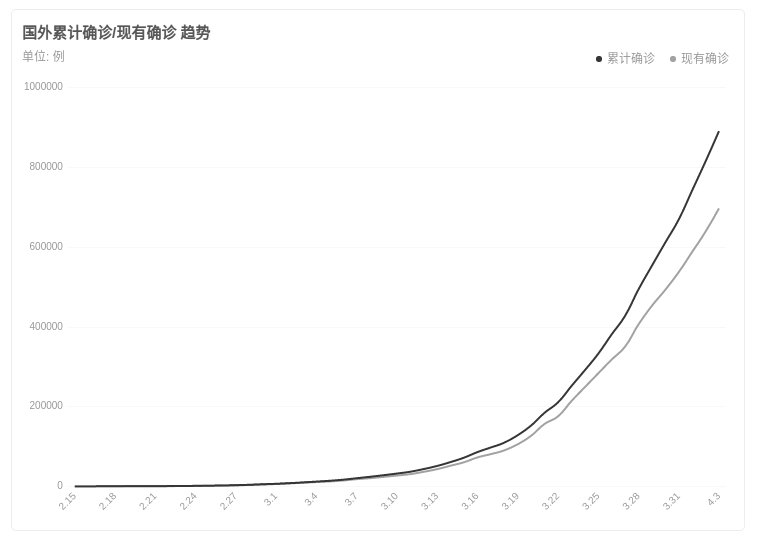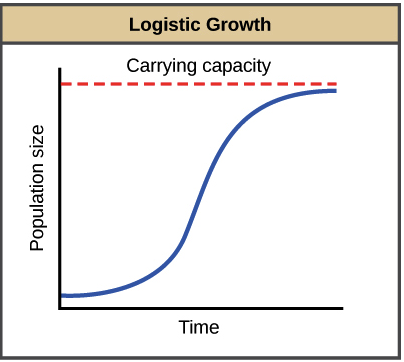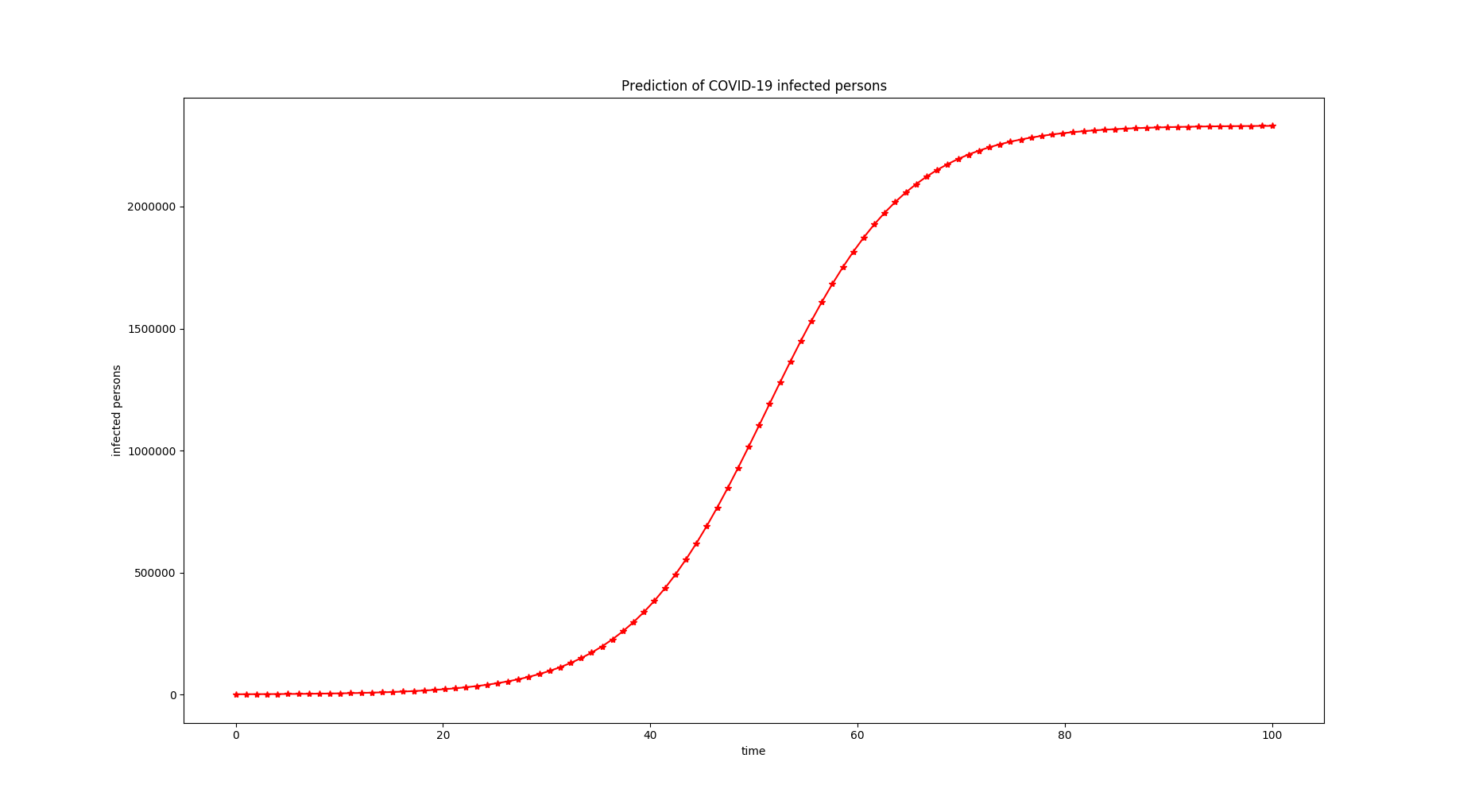Live as everything is a miracle or nothing is miracle$$y(t) = \frac{k}{1 + Ae^{-rt}}$$

$$y(t) = \frac{2332345.16}{1 + 2172.67e^{-0.15t}}$$0%